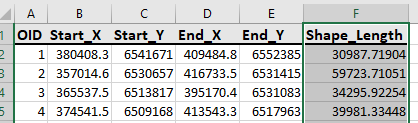# Get distance from X / Y coordinates from xls Excel file

537
7
06-22-2020 05:49 AMbyEsri Contributor

I have a xls Excel file with a startpoint and endpoint column each containing x and y coordinates and I need to get the distance between each start- and endpoint. The result (the calculated distance) should be added as new column into the file.

Can anybody help me out here with python code snippet or a workflow to achieve this?

Thanks in advance7 RepliesFrequent Contributor III

a² + b² = c²

Where c is the line between  a and b

Or simply  create lines from the coordinates and populate your spreadsheet with the shape length resulting from the linesbyEsri Contributor

Sorry I forgot an important information. The distance should be calculated via a Routing service (Street Map Premium or ArcGIS Online).Frequent Contributor III

Yep whole new level of complex.

We don't use routing services ourselves.

But what you could do is build the points in the gis and add a hyperlink using Google, Apple, or Bing maps to create the driving directions when you select the hyperlink.  It would at least give you the best route based on traffic.

I used just that when creating driving directions into Collector (before Collector had directions built in)

The field techs would click the hyperlink and it would pop up Google or Apple maps to give directions to the site they were going to.byEsri Contributor

My approach would look something like this:

I'd apply the tools in ArcGIS Pro and get the basic Python code from it, but I'm new to ArcGIS Pro and don't know which tools to use.Frequent Contributor III

just use the same tools you would use for ArcMapbyEsri Contributor

Here's the python code which takes an excel file with a startpoint and endpoint column each containing x and y coordinates as input and outputs an excel file with shape_length (distance) calculated in a new column.

Just change the variables' values, sheet name and the spatial_reference accordingly it should work.

``````import arcpy
import pandas as pd

excelTable = r"D:\temp\XY_Coordinates.xlsx"
csvTable = r"D:\temp\out_csv.csv"
outputFC = r"D:\temp\output.gdb\out_coordinates"
out_Excel = r"D:\temp\XY_Coordinates_output.xlsx"

arcpy.env.overwriteOutput = True

# Convert x and y coordinates into line
arcpy.XYToLine_management(
csvTable,
outputFC,
'Start_X',
'Start_Y',
'End_X',
'End_Y',
'NORMAL_SECTION',
'#',
"PROJCS['GDA_1994_MGA_Zone_50',GEOGCS['GCS_GDA_1994',DATUM['D_GDA_1994',SPHEROID['GRS_1980',6378137.0,298.257222101]],PRIMEM['Greenwich',0.0],UNIT['Degree',0.0174532925199433]],PROJECTION['Transverse_Mercator'],PARAMETER['False_Easting',500000.0],PARAMETER['False_Northing',10000000.0],PARAMETER['Central_Meridian',117.0],PARAMETER['Scale_Factor',0.9996],PARAMETER['Latitude_Of_Origin',0.0],UNIT['Meter',1.0]];-5120900 1900 10000;-100000 10000;-100000 10000;0.001;0.001;0.001;IsHighPrecision"
)

# Converting gdb feature class attribute to Excel
arcpy.TableToExcel_conversion(outputFC, out_Excel)‍‍‍‍‍‍‍‍‍‍‍‍‍‍‍‍‍‍‍‍‍‍‍‍‍‍‍‍‍‍‍‍‍‍‍‍‍‍‍‍‍‍‍‍‍‍‍‍‍‍‍‍‍‍``````

Here's a screenshot of the output:byEsri Contributor

Thanks Mehdi Pira‌ !

This is definitely a way in the right direction. Although I forgot to mention, that I need to calculate the distances based on a street network (SMP) and the results from your approach are direct lines between the points, right?

Thank you anyway for the "Excel Hint" this was also very helpful!

If anybody could suggest if I should rather try to implement a solution with ODCM Matrix or CF, maybe just a Route? The only thing we need is the distance in km.